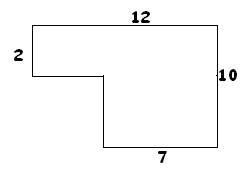1. /
2. CBSE
3. /
4. Class 07
5. /
6. Perimeter and Area worksheet...

# Perimeter and Area worksheet for class 7### myCBSEguide App

Download the app to get CBSE Sample Papers 2023-24, NCERT Solutions (Revised), Most Important Questions, Previous Year Question Bank, Mock Tests, and Detailed Notes.

## Perimeter and Areaworksheet for class 7 Important Topics

• 11.1 Triangles
• 11.2 Area of a parallelogram & triangle
• 11.3 Circles : Circumference & Area
• 11.4 Perimeter of Polygons
• 11.5 Conversion of units and its applications## Some important Facts about Perimeter and Areaworksheet for class 7

1. Perimeter is the distance around a closed figure whereas area is the part of plane occupied by the closed figure.
2. We have learnt how to find perimeter and area of a square and rectangle in the earlier class. They are: (a) Perimeter of a square = 4 × side (b) Perimeter of a rectangle = 2 × (length + breadth) (c) Area of a square = side × side (d) Area of a rectangle = length × breadth
3. Area of a parallelogram = base × height

## NCERT class 7Mathematics Solved Worksheets

• Chapter 1 – Integers
• Chapter 2 – Fractions and Decimals
• Chapter 3 – Data Handling
• Chapter 4 – Simple Equations
• Chapter 5 – Lines and Angles
• Chapter 6 – Practical Geometry
• Chapter 7 – The Triangle and its Properties
• Chapter 8 – Congruence of triangles
• Chapter 9 – Comparing Quantities
• Chapter 10 – Rational numbers
• Chapter 11 – Perimeter and Area
• Chapter 12 – Algebraic Expressions
• Chapter 13 – Exponents and Powers
• Chapter 14 – Symmetry
• Chapter 15 – Visualizing Solid Shapes

## SQUARES AND RECTANGLES

Ayush and Deeksha made pictures. Ayush made his picture on a rectangular sheet of length 60 cm and breadth 20 cm while Deeksha made hers on a rectangular sheet of length 40 cm and breadth 35 cm. Both these pictures have to be separately framed and laminated. Who has to pay more for framing, if the cost of framing is  3.00 per cm? If the cost of lamination is  2.00 per cm2 , who has to pay more for lamination?

For finding the cost of framing, we need to find perimeter and then multiply it by the rate for framing. For finding the cost of lamination, we need to find area and then multiply it by the rate for lamination

To download Printable worksheets for class 7 Mathematics and Science; do check myCBSEguide app or website. myCBSEguide provides sample papers with solution, test papers for chapter-wise practice, NCERT solutions, NCERT Exemplar solutions, quick revision notes for ready reference, CBSE guess papers and CBSE important question papers. Sample Paper all are made available through the best app for CBSE students and myCBSEguide website.### Test Generator

Create question paper PDF and online tests with your own name & logo in minutes.### myCBSEguide

Question Bank, Mock Tests, Exam Papers, NCERT Solutions, Sample Papers, Notes